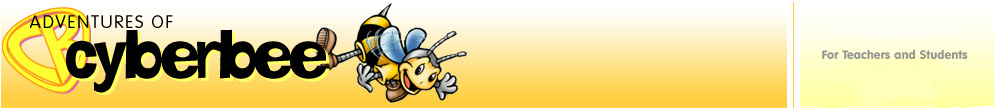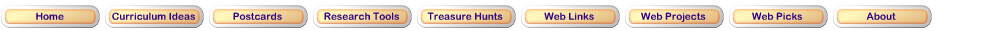Measurement Standard for Grades 3-5

Understand measurable attributes of objects and the units, systems, and processes of measurement
• understand such attributes as length, area, weight, volume, and size of angle and select the appropriate type of unit for measuring each attribute;
• understand the need for measuring with standard units and become familiar with standard units in the customary and metric systems;
• carry out simple unit conversions, such as from centimeters to meters, within a system of measurement;
• understand that measurements are approximations and how differences in units affect precision;

Apply appropriate techniques, tools, and formulas to determine measurements
• develop strategies for estimating the perimeters, areas, and volumes of irregular shapes;
• select and apply appropriate standard units and tools to measure length, area, volume, weight, time, temperature, and the size of angles;
• select and use benchmarks to estimate measurements;

Try these Websites to practice your measuring skills.Measuring in Inches and Centimeters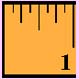FunBrain will show you a ruler with a red bar above it. Select the answer that states the length of the red bar. Practice with metric or customary units.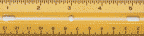Customary Units
 Click on the Ruler and print it. Then use it to measure the activities listed below.Use a sheet of paper to do the following activities: Estimate the length of your right foot.What instrument will you use to measure your right foot? Measure your right foot. How will you label your answer?How long is your right foot? How close is this to your estimate?Are both of your feet the same size? What measuring instruments can you use to determine your answer?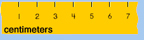Metric UnitsE-Lab Activity Click on Try This and go to Estimating Metric Math.Drag the ruler to the line. Measure the length of the line. Type in the length in centimeters. Press enter or return.Practice Metric Measurement. Print the recording sheet and see how you do at estimating lengths.Measuring in Pounds and KilogramsICT Games Do you know your kilograms from your pounds? First visit Mostly Postie. Drag the packages onto the empty scales and type in how much it weighs. Click on the check button to see if your answer is correct.Classroom Activity Estimate what you think an item weighs. Then weigh it on a set of scales. How close was your estimate?Measuring Temperature in Fahrenheit and CelsiusClassroom Activity Print the worksheet from TLSBooks and find the temperature inside and out.Math is Fun Drag the slider on the thermometer at the Math is Fun site to see a comparison between the Fahrenheit and Celsius Scales.Updated March 14, 2017
© 1996 - 2009 Linda C. Joseph